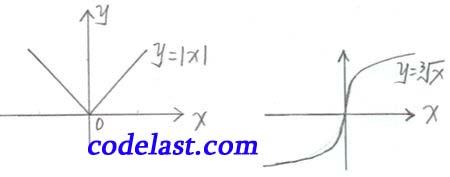## [原创]高等数学笔记(24)

【前言】

【正文】

$y = \sqrt{x}$ ，自变量在 $x = 0$ 点有增量 $\Delta x$ ，则 $\Delta y = \sqrt{{0 + \Delta x}} - \sqrt{0} = \sqrt{{\Delta x}}$

$\mathop {\lim }\limits_{\Delta x \to 0} \frac{{\Delta y}}{{\Delta x}} = \mathop {\lim }\limits_{\Delta x \to 0} \frac{{\sqrt{{\Delta x}}}}{{\Delta x}} = \mathop {\lim }\limits_{\Delta x \to 0} \frac{1}{{{{(\Delta x)}^{\frac{2}{3}}}}} = \infty$

$y = \sqrt {{x^2}} = \left| x \right| = \left\{ {\begin{array}{*{20}{c}}{x,x \ge 0}\\{ - x,x < 0}\end{array}} \right.$ ，易证 $y = \left| x \right|$$x = 0$ 点连续（这里就不详细写了）

$\Delta y = \left| {0 + \Delta x} \right| - \left| 0 \right| = \left| {\Delta x} \right| = \left\{ {\begin{array}{*{20}{c}}{\Delta x,\Delta x > 0}\\{ - \Delta x,\Delta x < 0}\end{array}} \right.$
$x = 0$ 处的右导数 ${{f'}_ + }(0) = \mathop {\lim }\limits_{\Delta x \to {0^ + }} \frac{{\Delta y}}{{\Delta x}} = \mathop {\lim }\limits_{\Delta x \to {0^ + }} \frac{{\Delta x}}{{\Delta x}} = 1$
$x = 0$ 处的左导数 ${{f'}_ - }(0) = \mathop {\lim }\limits_{\Delta x \to {0^ - }} \frac{{\Delta y}}{{\Delta x}} = \mathop {\lim }\limits_{\Delta x \to {0^ - }} \frac{{ - \Delta x}}{{\Delta x}} = - 1$1. 常数 $C$$f(x) \equiv C, - \infty < x < + \infty$

$y = f(x) \equiv C,\;\forall x \in ( - \infty , + \infty )$
$\Delta y = f(x + \Delta x) - f(x) = C - C = 0$
$f'(x) = \mathop {\lim }\limits_{\Delta x \to 0} \frac{{\Delta y}}{{\Delta x}} = \mathop {\lim }\limits_{\Delta x \to 0} \frac{0}{{\Delta x}} = 0$

2. 幂函数 $y = f(x) = {x^\alpha }$$\alpha$ 为实常数）

$\alpha = n(n \in N)$ 时，有 $\Delta y = f(x + \Delta x) - f(x) = {(x + \Delta x)^n} - {x^n}$

$\Delta y = \left[ {{x^n} + n{x^{n - 1}}\Delta x + \frac{{n(n - 1)}}{{2!}}{x^{n - 2}}{{(\Delta x)}^2} + \cdots + {{(\Delta x)}^n}} \right] - {x^n}$
$= n{x^{n - 1}}\Delta x + \frac{{n(n - 1)}}{{2!}}{x^{n - 2}}{(\Delta x)^2} + \cdots + {(\Delta x)^n}$

（注：从第二项开始，每一项的极限均为0）

$\alpha$ 为任何实常数时， $({x^\alpha })' = \alpha {x^{\alpha - 1}}$ ，这个结论以后再证明。

3. 正弦、余弦函数 $y = f(x) = \sin x,f(x) = \cos x$

$y = \sin x,\; - \infty < x < + \infty$
$\forall x \in ( - \infty , + \infty )$ ，自变量有增量 ${\Delta x}$ ，函数 $y = \sin x$ 的增量 $\Delta y = \sin (x + \Delta x) - \sin x = 2\sin \frac{{\Delta x}}{2}\cos (x + \frac{{\Delta x}}{2})$
（注：三角函数的和差化积公式）

（注： $\mathop {\lim }\limits_{\Delta x \to 0} \frac{{2\sin \frac{{\Delta x}}{2}}}{{\Delta x}} = 1$ 是重要极限之一； $y = \cos x$ 是连续函数，因此 $\mathop {\lim }\limits_{\Delta x \to 0} \cos (x + \frac{{\Delta x}}{2})$ 的极限号可以放进去）

$y = \cos x,\; - \infty < x < + \infty$
$\forall x \in ( - \infty , + \infty )$$\mathop {\lim }\limits_{\Delta x \to 0} \frac{{\Delta y}}{{\Delta x}} = \mathop {\lim }\limits_{\Delta x \to 0} \frac{{\cos (x + \Delta x) - \cos x}}{{\Delta x}} = \mathop {\lim }\limits_{\Delta x \to 0} \frac{{ - 2\sin \frac{{\Delta x}}{2}\sin \left( {x + \frac{{\Delta x}}{2}} \right)}}{{\Delta x}}$
$= - \mathop {\lim }\limits_{\Delta x \to 0} \frac{{\sin \frac{{\Delta x}}{2}}}{{\frac{{\Delta x}}{2}}} \cdot \mathop {\lim }\limits_{\Delta x \to 0} \sin \left( {x + \frac{{\Delta x}}{2}} \right) = - \sin x$

4. 对数函数 $y = f(x) = {\log _a}x\;(a > 0,a \ne 1)$
$y = {\log _a}x,\;0 < x < + \infty$
$\forall x \in (0, + \infty )$ ，设自变量 $x$ 有增量 ${\Delta x}$ ，函数对应的增量：
$\Delta y = {\log _a}(x + \Delta x) - {\log _a}x = {\log _a}\left( {\frac{{x + \Delta x}}{x}} \right) = {\log _a}\left( {1 + \frac{{\Delta x}}{x}} \right)$

$= \frac{1}{x} \cdot {\log _a}\left[ {\mathop {\lim }\limits_{\Delta x \to 0} {{\left( {1 + \frac{{\Delta x}}{x}} \right)}^{\frac{x}{{\Delta x}}}}} \right] = \frac{1}{x} \cdot {\log _a}e = \frac{1}{x} \cdot \frac{1}{{\ln a}} = \frac{1}{{x\ln a}}$
（注： $\mathop {\lim }\limits_{\Delta x \to 0} {\left( {1 + \frac{{\Delta x}}{x}} \right)^{\frac{x}{{\Delta x}}}} = e$ 是重要极限之一，即 $\mathop {\lim }\limits_{\alpha \to 0} {(1 + \alpha )^{\frac{1}{\alpha }}} = e$

$(\ln x)' = \frac{1}{{x\ln e}} = \frac{1}{x}$

${\left( C \right)^\prime } = 0$
${\left( {{x^\alpha }} \right)^\prime } = \alpha {x^{\alpha - 1}}$
${\left( {\sin x} \right)^\prime } = \cos x$
${\left( {\cos x} \right)^\prime } = - \sin x$
${\left( {{{\log }_a}x} \right)^\prime } = \frac{1}{{x\ln a}}$
${\left( {\ln x} \right)^\prime } = \frac{1}{x}$

（第24课完）

➤➤ 版权声明 ➤➤